# How to extract formula from Excel using Alteryx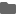2021.06.11

(日本語はこちらから)

## Introduction:

Alteryx is a convenient data-prep tool which can import data from a variety of files. Take the case of Excel, the input can be from a single or multiple files having the target data distributed in one or more excel-sheets. Those files can be located in various directories and can have the same or different schema.

However, these examples mentioned above would just import the data and not the underlying formula which was used to compute the data values. A visual example is shown here: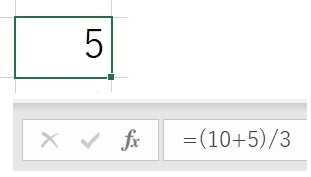By default, Alteryx would import the data value “5” and not the underlying formula “=(10+5)/3” which was used to calculate it. Depending on the requirements, sometimes, the user may want to import the formula to understand the logic or for debugging etc.

This post will explain how to extract the formula from excel files.

## How to achieve:

Let us consider an excel file with the following data, note that columns A, B and C hold the alpha-numeric values, while column D is a calculation B/C*100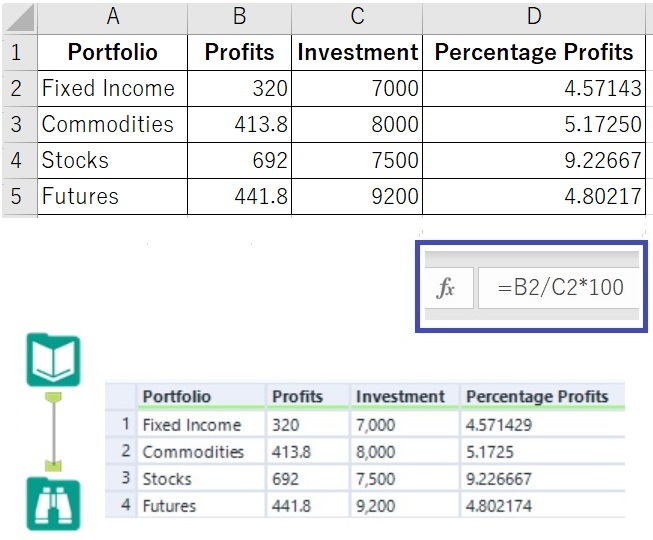When we read an excel file in Alteryx we just import its data values, so in order to extract the formula, the trick here is to treat it as if it was a zip file. In the input tool configuration, choose “File Format” as Zip (*.zip) and click on the “Table or Query” and select the “worksheets” sub-folder as shown below.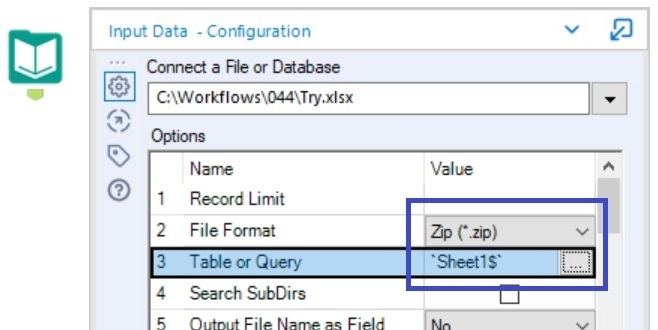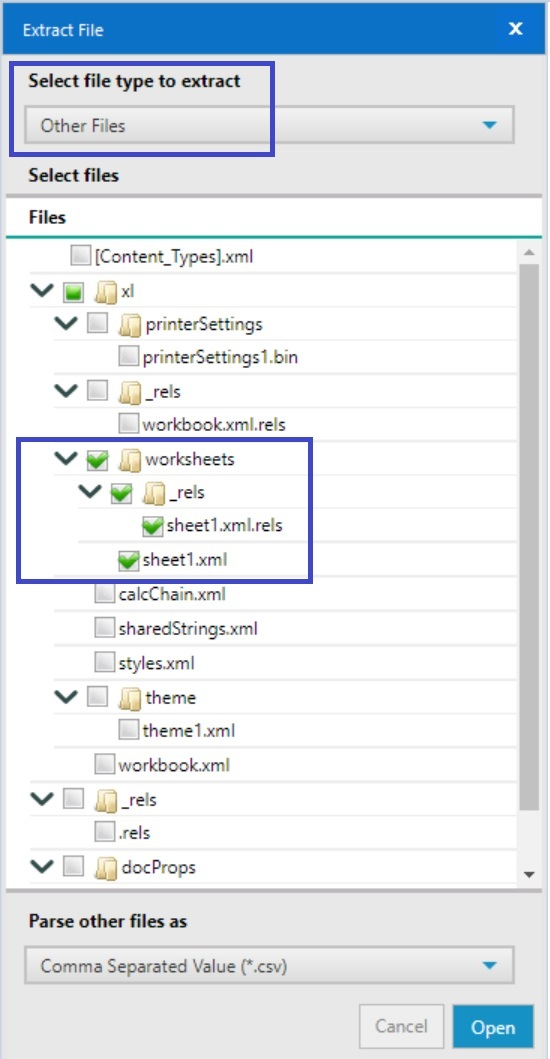Further configure the input tool so that the delimiters are set to “\n”, “First Row Contains Field Names” is deselected and “Field Length” is set to a higher value to accommodate all the input characters as shown below.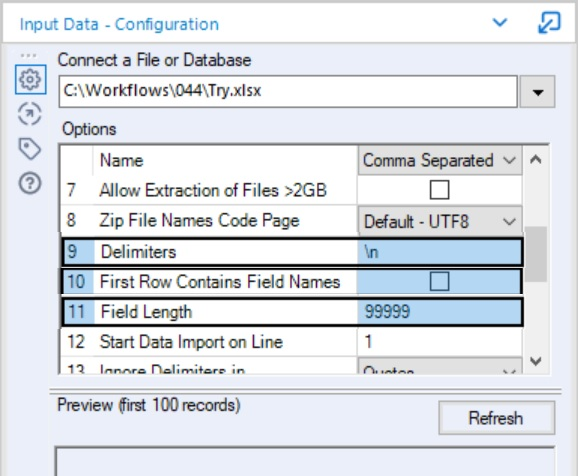Next, add a sampling tool to just pick up the last row from the data.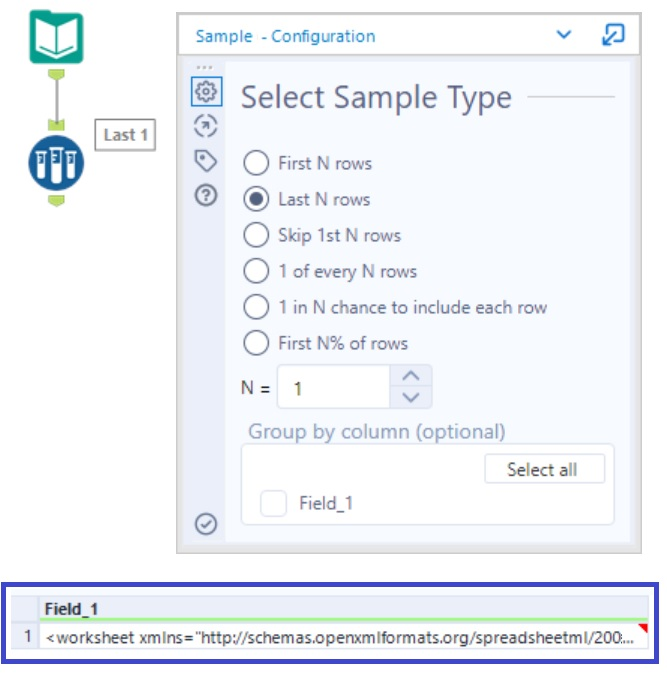Finally to extract the information from the input file, use the XML Parse tool with the following configuration.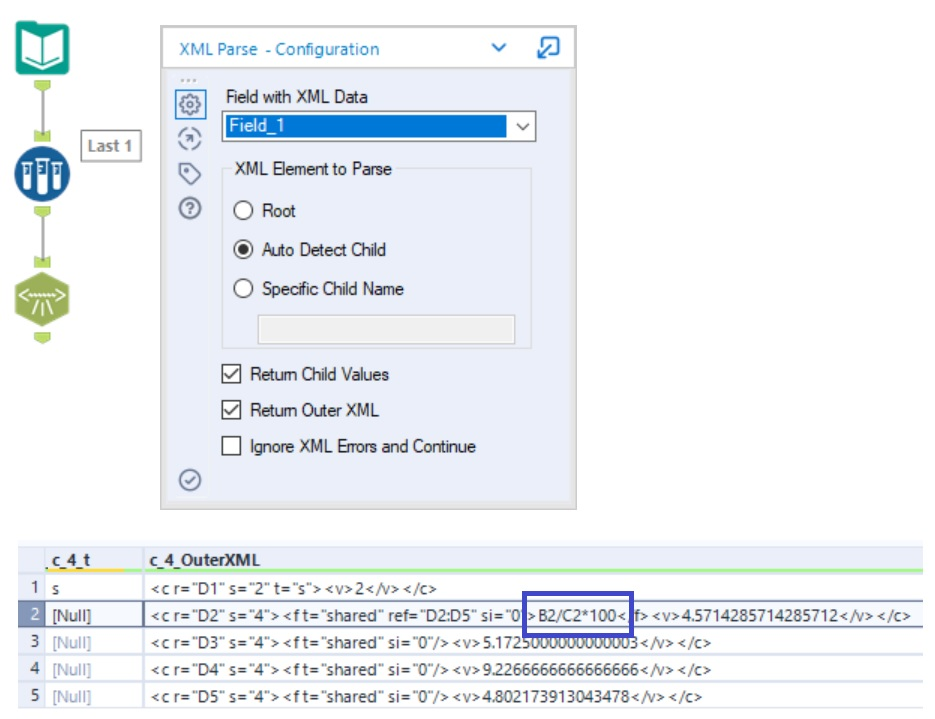The formula can be found in the “n”th outer XML, where “n” is the column number of excel where the formula exists. In this case the formula is present in the 4th column, so the result can be extracted from c_4_OuterXML. Or it can also be found in the row_OuterXML column.

Thus with the help of some workaround, it is possible to extract the formulae from an existing excel file.

## Summary

With the help of Alteryx, it is possible to extract the formula which was used to create a calculation in an excel file.

### Alteryxの導入なら、クラスメソッドにおまかせください